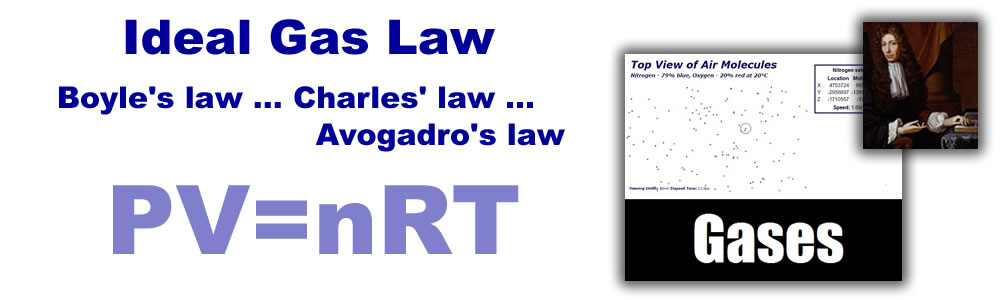# The Ideal Gas LawThe Ideal Gas Law states that at constant temperature for a fixed mass, the absolute pressure and the volume of a gas are inversely proportional. To model the normal air we breath at 20°C we are dealing with free flowing molecules travelling in the range of a few nanometers per picosecond. We chose a femtometer (10^-15) gridscale, with timesteps every 1/10 of a picosecond (10^-12). With these settings, we can watch molecules move across the screen at speeds around one or two nanometers per picosecond within a 10 x 10 x 10 nanometer cube with 6 digits of accuracy.

To model the air we breath, first calculate the molecular speed depending upon the molecular mass and the temperature. The air we breath is made up of mostly oxygen O2 (20%) and nitrogen N2 (79%). Oxygen floats around as pairs of oxygen molecules and and the nitrogen also floats around in pairs. Oxygen’s 8 protons and neutrons gives it a weight of 16 atomic mass units (amu), and a pair would be 32 amu. Nitrogen’s proton count of 7, leads to 14 amu’s and as pair is 28 amu’s. This makes the average air molecule around 29 amu’s.

#### We need the average speed of the molecules

In statistical mechanics the Ideal Gas Law is derived from first principles where the pressure on the outside of the container is cause by the collisions of moving molecules within. More molecules within the same space means a higher pressure. A higher temperature means each molecule is moving faster which

Ideal Gas Law: P*V = nRT = N*kB*T

where P is the absolute pressure of the gas, n is the amount of gas (number of moles, R is the universal gas constant, N is the number of molecules in the given volume V, kB is the Boltzmann constant relating temperature and energy, and T is the absolute temperature.

From this, the average speed can be calculated as √(3RT/M), where M is the mass of the molecule.  For gas constant R = 8314, temperature T= 293.15K  (T= 20C) and mass M= 29 amu the average speed is √(3*8314*293) /29) = 502 meters/second (1807km/hr or 1122mi/hr)  = 0.5 nanometers/picosecond.

#### We need to know how many molecules in a given space

Rewriting the gas law to calculate how many partiles, we get N=P*V/kB*T. At room temperature, T=20C (293K), the average presure P = 101000Pa (pascal = N/M^2 = J/M^3). The volumn of a 10 x 10 x 10 nanometer cavity V = (10^-8)^3 = 10^-24 and the Boltzman constant kB = 1.38×10^-23 J/K.  Substituting,  N=P*V/kB*T = (101000*10^-24) / (1.38*10^-23^293) = 25 molecules.

And to quickly double check this using the normal density of air, 1.223 kg/m^3, we convert kilograms to atomic mass units and meter cubed to nanometers cubed and we get density of air = 1.225*6.022*10^26/(10^9)^3 = 0.738 amu/nm. In a 10 x 10 x 10 nanometer cube, there would be 1000*0.738 amu = 738 amu = 25.4 molecules with an average amu of 29.

In simple terms, the air we breath contains about 25 molecules in a 10 nanometer cube traveling around at over 1100 miles/hour.